MORE IN Analog Electronics - 1
MU Electronics and Telecom Engineering (Semester 3)
Analog Electronics - 1
December 2014
Total marks: --
Total time: --
INSTRUCTIONS
(1) Assume appropriate data and state your reasons
(2) Marks are given to the right of every question
(3) Draw neat diagrams wherever necessary

Attempt any five:
1 (a) The PNP transistor shown in Fig a has ?=50. Find the value of Rc to obtain Vc=+5V. What happens if transistor is replaced with another having ?=100.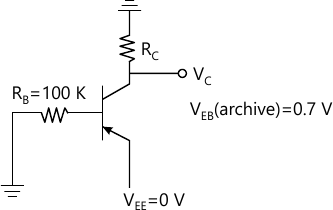5 M
1 (b) Draw small signal model of JFET and explain significance of each parameter.
5 M
1 (c) Why common collector amplifier is used as buffer. Why buffers are required.
5 M
1 (d) Write down current equation of diode and explain significance of each parameters.
5 M
1 (e) For the circuit shown in Fig le. Find IDS and VDS if VRS=1.5V.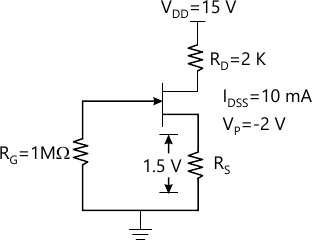5 M
1 (f) Compare Collpit's and Clapp's oscillator.
5 M

2 (a) Explain working of n-channel eMOSFET with the help output characteristics, showing clearly effect of channel length modulation. Give equation of drain current in linear and saturation current along with conditions.
10 M
2 (b) Design JFET circuit with voltage divider biasing as shown in Fig 2b with JFET parameters IDSS=12mA, VF=3.5V and ?=0. Let R1+R2=100K, IDSQ=5mA. And VDSQ=5V.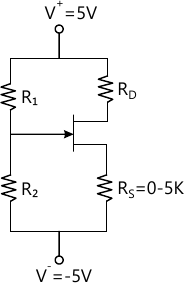10 M

3 (a) Draw circuit diagram of common emitter amplifier with voltage divider bias with bypassed emitter resistance and derive expression for voltage gain, current gain, input resistance, output resistance using hybrid-?- model which includes early effect.
10 M
3 (b) In n-channel E-MOSFET
i) Substrate doping NA=1016 cm-3.
ii) Poly=silicon Gae doping ND=1020cm-3
iii) Gate oxide thickness tox=0.5 ?m
iv) Oxide positive charge interface density=4&time;1010 cm-2
v) Charge of electron=1.6×10-19 col
vi) Permittivity of free space &insin;0=8.85&time;10-14 F/cm.
vii) Dielectric constant of Si=11.9

viii) Dielectric constant of Si02=3.9
Find zero bias threshold voltage (VTO)
10 M

4 (a) Explain the working of Wien-Bridge Oscillator. Derive the expression for frequency of Oscillation and the value of gain required for sustained oscillation.
10 M
4 (b) For the circuit shown i Fig 4b, assume ?=100. i) Find Thevenin's equivalent voltage VTH and resistance RTH for base circuit ii) Determine ICQ and VCEQ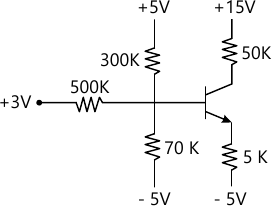10 M

5 (a) Draw a required diode clamper circuit to generate the output VD to from the input Vt as shown in Fig 5a if ii) V?=0.7V. Where V? is cut-in voltage of diode.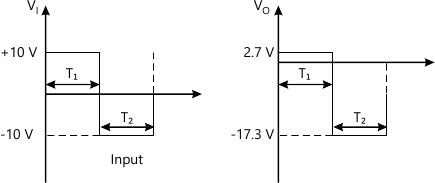10 M
5 (b) What are different biasing techniques used to bias D-MOSFET and E-MOSFET. Explain with the help of appropriate circuit diagrams.
10 M

6 (a) Hybrid-? model of BJT
5 M
6 (b) Twin-T oscillator
5 M
6 (c) AC and DC load line
5 M
6 (d) MOS capacitor.
5 M

More question papers from Analog Electronics - 1